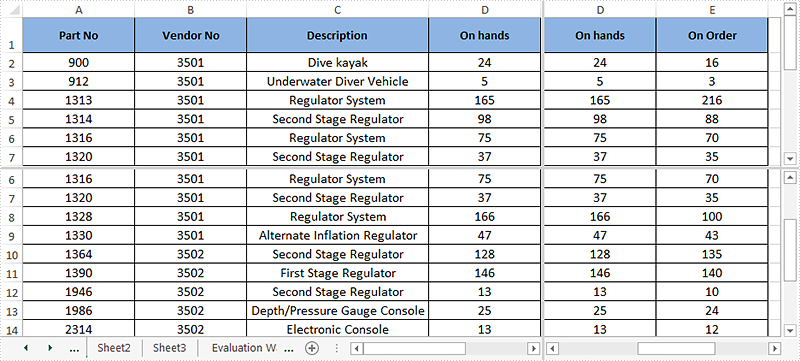# How to Split Excel Worksheet into Multiple Panes in C#

Excel enables us to split an excel worksheet into two or four independent panes. After splitting up the window into panes, we can use the horizontal and vertical scroll bars to view and compare data in different parts of the same worksheet. This article demonstrates how to vertical and horizontal split an excel worksheet into four panes programmatically using Spire.XLS.

Detail steps:

Step 1: Instantiate a Workbook instance and load the excel document.

```Workbook workbook = new Workbook();
```

Step 2: Get the first worksheet.

```Worksheet sheet = workbook.Worksheets;
```

Step 3: Split the worksheet horizontally and vertically.

```sheet.FirstVisibleColumn = 3;

sheet.FirstVisibleRow = 5;

sheet.VerticalSplit = 110;

sheet.HorizontalSplit = 100;
sheet.ActivePane = 1;
```

Step 4: Save the excel document.

```workbook.SaveToFile("Output.xlsx", ExcelVersion.Version2013);
```

Screenshot after splitting:Full codes:

```using Spire.Xls;

namespace Split_Panes
{
class Program
{
static void Main(string[] args)
{
//Load the excel document
Workbook workbook = new Workbook();

//Get the first worksheet
Worksheet sheet = workbook.Worksheets;

//Vertical and horizontal split the worksheet into four panes

sheet.FirstVisibleColumn = 3;

sheet.FirstVisibleRow = 5;

sheet.VerticalSplit = 110;

sheet.HorizontalSplit = 100;

sheet.ActivePane = 1;

//Save the excel document
workbook.SaveToFile("Output.xlsx", ExcelVersion.Version2013);

workbook.Dispose();
}
}
}
```